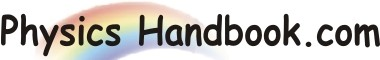HOME TOPICS DEFINITIONS TABLES LAWS INVENTIONS EXPERIMENTS QUIZ VIDEOS
 Newton's First Law A body persists its state of rest or of uniform motion unless acted upon by an external unbalanced force. It can also be stated as a body that is acted on by no net force moves at a constant velocity (which may be zero) and zero acceleration. The net force on an object is the vector sum of all the forces acting on the object. Newton's first law says that if this sum is zero, the state of motion of the object does not change. Essentially, it makes the following two points: - An object that is not moving will not move until a net force acts upon it. - An object that is in motion will not change its velocity (accelerate) until a net force acts upon it. Newton's First Law of Motion states that in order for the motion of an object to change, a force must act upon it, a concept generally called inertia.